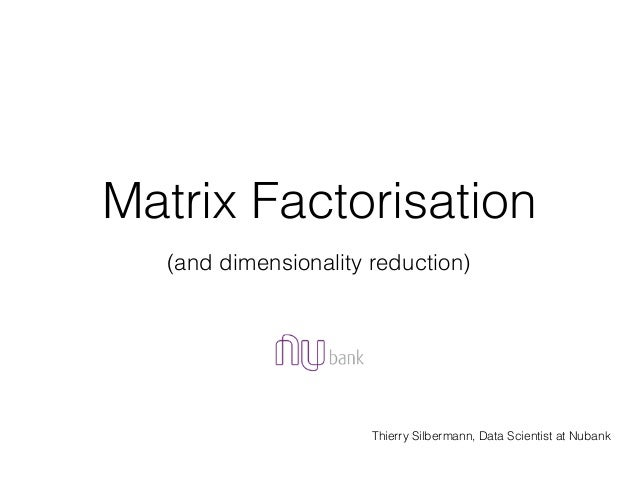Successfully reported this slideshow.Upcoming SlideShare
×

# Matrix Factorisation (and Dimensionality Reduction)

3,772 views

Published on

Slides from the "Matrix Factorisation" presentation by Thierry Silbermann at the Sao Paulo Machine Learning Meetup.

Published in: Technology
• Full Name
Comment goes here.

Are you sure you want to Yes No• Dating direct: ❶❶❶ http://bit.ly/2F90ZZC ❶❶❶

Are you sure you want to  Yes  No
• Dating for everyone is here: ❤❤❤ http://bit.ly/2F90ZZC ❤❤❤

Are you sure you want to  Yes  No

### Matrix Factorisation (and Dimensionality Reduction)

1. 1. Matrix Factorisation Thierry Silbermann, Data Scientist at Nubank (and dimensionality reduction)
2. 2. What is Matrix Factorisation? • For some domain, we have matrix that are: very ‘big’ sparse and don’t have any order • Factoring it yield a set of: More manageable compact and ordered matrices
3. 3. Matrix Factorisation / Decomposition • Singular Value Decomposition (SVD) • Principal Component Analysis (PCA) • Non-negative Matrix Factorisation (NMF) • LU/QR/Cholskey decomposition • etc…
4. 4. SVD (Singular Value Decomposition) • The singular value decomposition of an m × n matrix M is a factorisation of the form : M = UΣV∗, where U is an m × m unitary matrix, Σ is an m × n rectangular diagonal matrix with non-negative real numbers on the diagonal (known as the singular values of M), and V∗ (the transpose of V) is an n × n real or complex unitary matrix. The m columns of U and the n columns of V are called the left- singular vectors and right-singular vectors of M, respectively.
5. 5. Limitation SVD • One of the most general algorithm for decomposition. SVD is an amelioration from eigenvalue decomposition (was only used with n x n matrix) • Didn’t really reduce the space use to store our data. • From a m x n (M) matrix, we end up with m x m (U), m x n (Σ) and n x n (V) matrices…
6. 6. Matrix Factorisation • Using Non-Negative Matrix Factorisation V ≈ WH • V, W and H are all non-negative • V is a n x m matrix • W is a n x r matrix, H is a r x m matrix • and r ≪ min(m, n)
7. 7. How to decompose? • Minimize with respect to W and H, subject to the constraints W, H ≥ 0. • Multiplication Update Algorithm • Alternating Least Square Algorithm
8. 8. Matrix Factorisation
9. 9. Toy example http://www.quuxlabs.com/blog/2010/09/matrix-factorization-a-simple-tutorial-and-implementation-in-python/
10. 10. Back to the math • We have a matrix of ratings we want to approximate: • We need to construct P and Q by minimising: • We have an optimisation problem: • Update are then give by: http://www.quuxlabs.com/blog/2010/09/matrix-factorization-a-simple-tutorial-and-implementation-in-python/
11. 11. Easy to implement (for toy example) http://www.quuxlabs.com/blog/2010/09/matrix-factorization-a-simple-tutorial-and-implementation-in-python/
12. 12. Easy to implement http://www.quuxlabs.com/blog/2010/09/matrix-factorization-a-simple-tutorial-and-implementation-in-python/
13. 13. Example: Recommend given names to user of the name search engine ”Nameling*”
14. 14. Example: Recommend given names to user of the name search engine ”Nameling*”
15. 15. Example: Recommend given names to user of the name search engine ”Nameling*” *http://nameling.net/